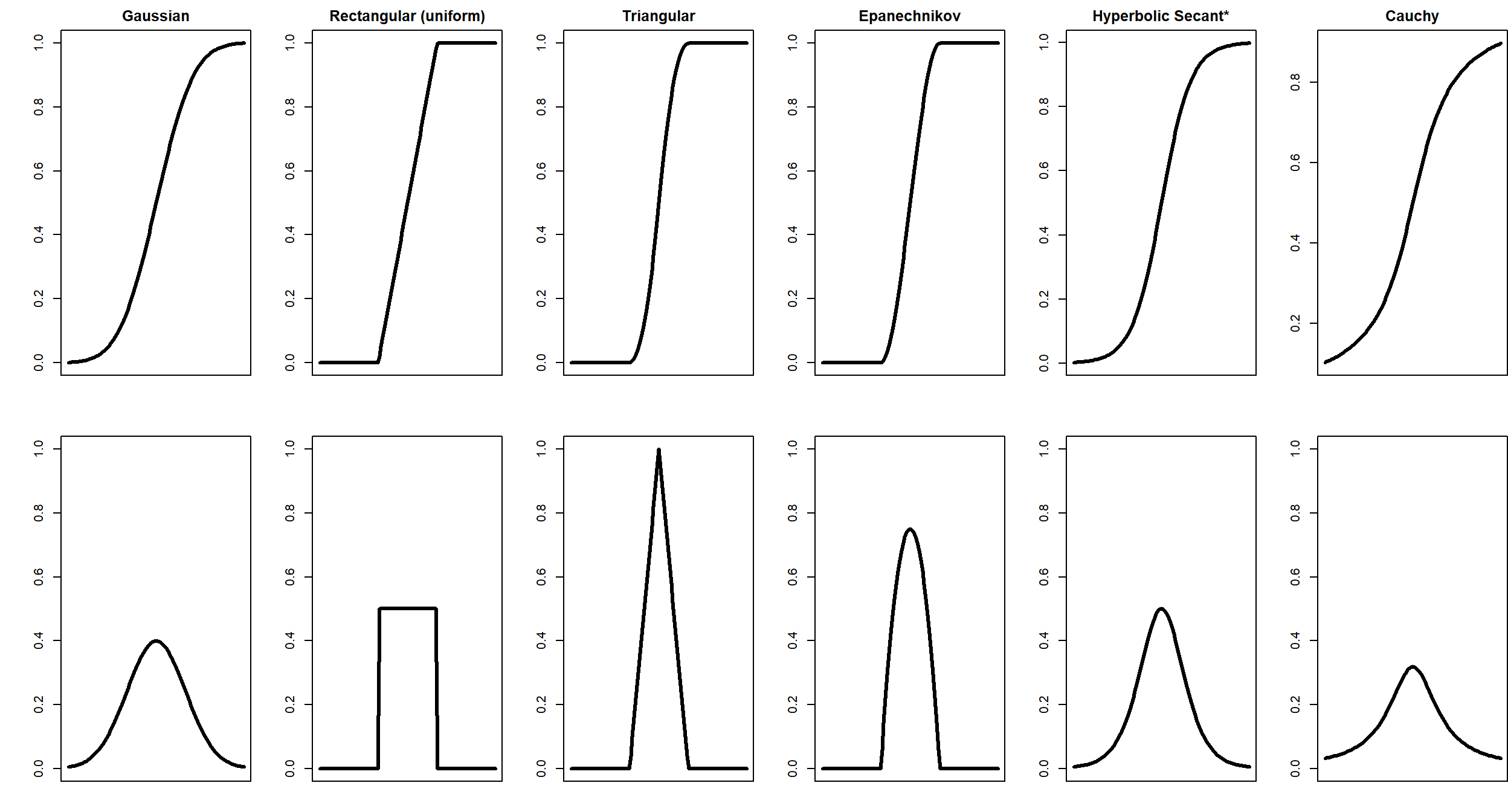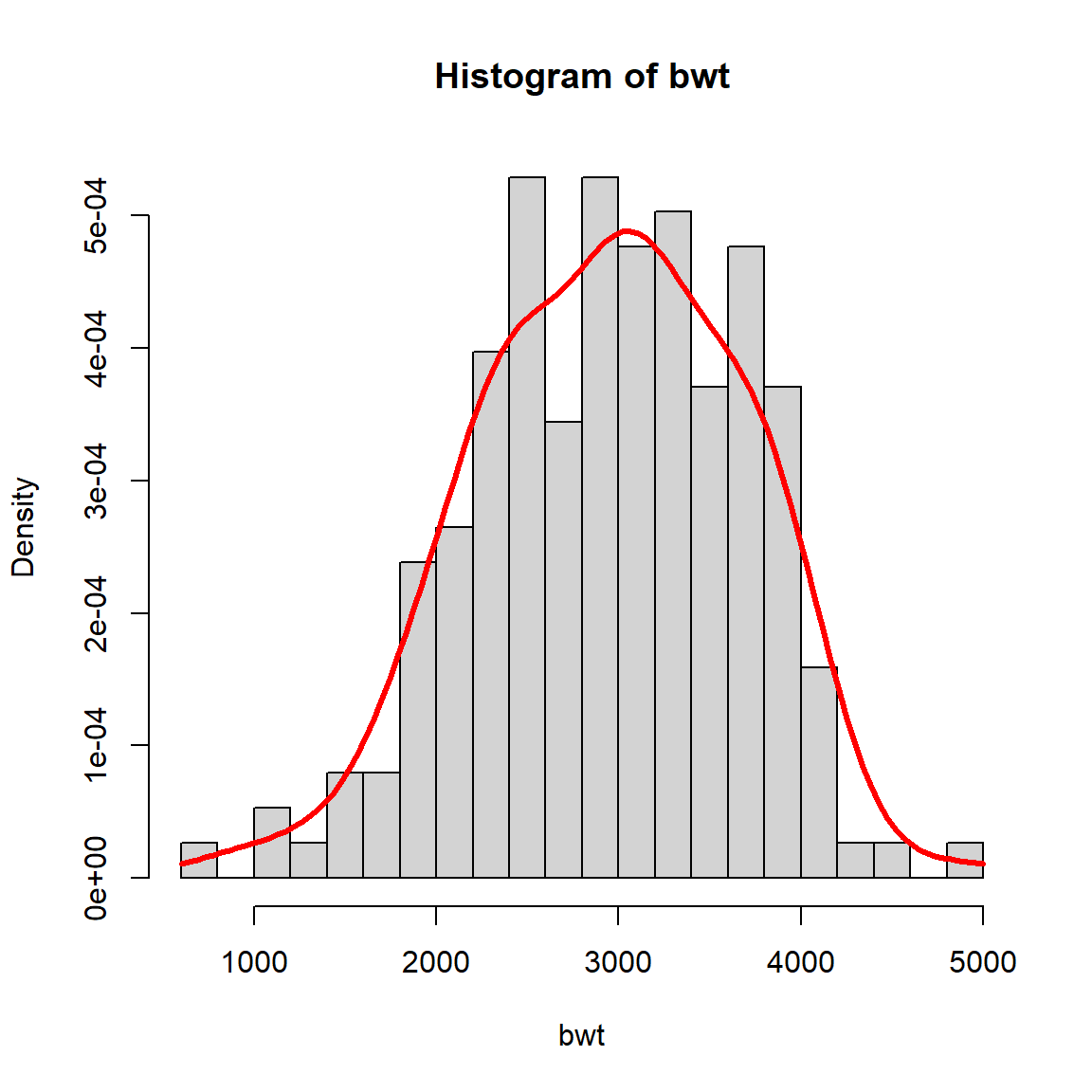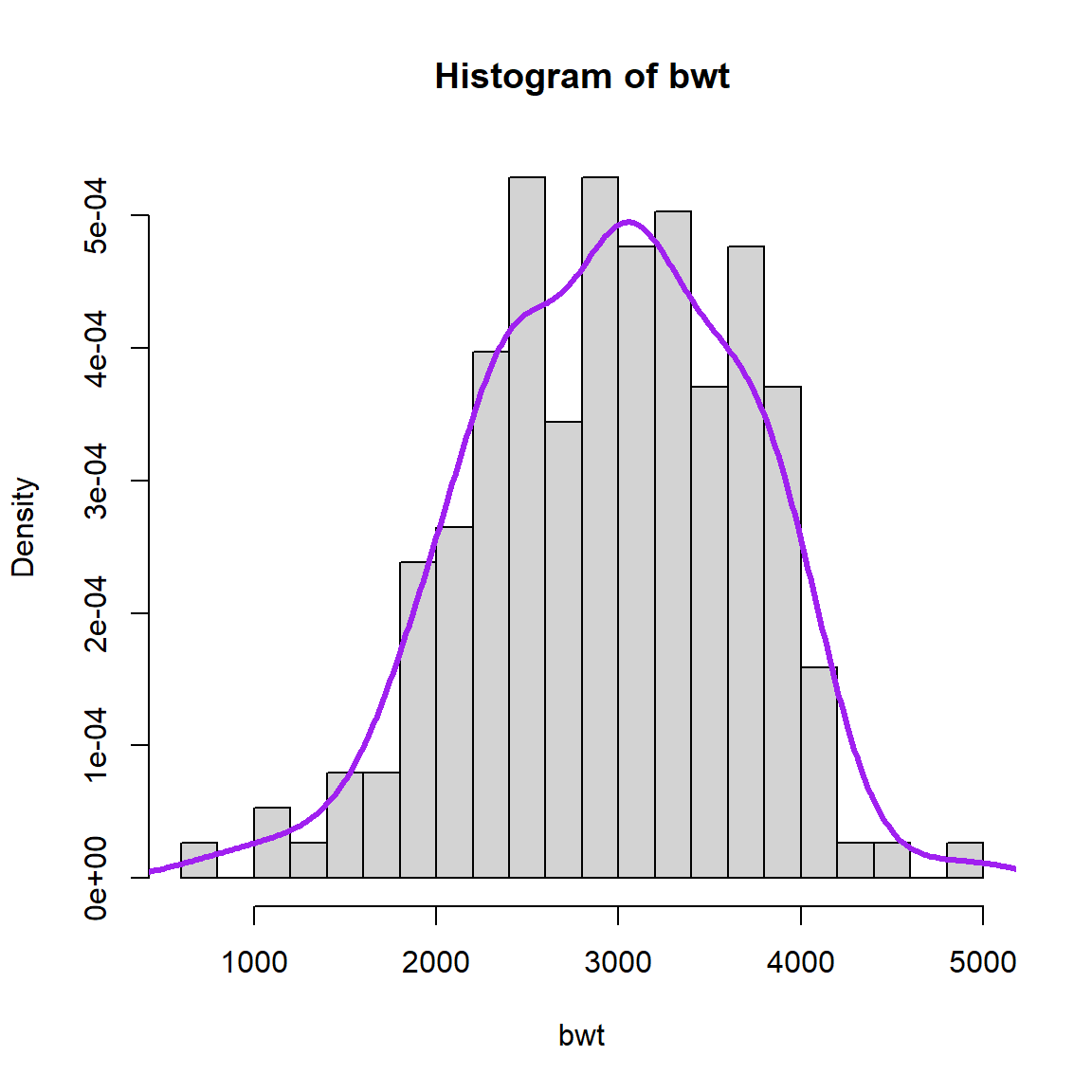# CDFs, PDFs, and Estimation with Kernels (Part 2)

## Recall

In the previous modules, we learned about

• The Empirical Cumulative Distribution Function (eCDF) as an estimate of the unknown CDF of a continuous random variable.
• Estimating a smooth CDF from data by
• Choosing from a family of smooth distributions
• Choosing from a mixture of smooth distributions
• Using a smoothing kernel

## In this module, we will shift from estimating the CDF to estimating the PDF

Call back: What is a PDF? What are the inputs? What are the outputs? Can you give an example of a PDF? What is the connection between a CDF and a PDF? (Can you verbalize your answers?)

We continue with the birth weight data.

## Selecting from a family of smooth distributions.

In a previous module, you “fit-by-eye” a normal, gamma, and mixture of two normals model. You are going to revisit that same data again, this time choosing the parameters while looking at both the resulting CDF and PDF.

Navigate here (link). Note: Typically CDFs and PDFs are on very different scales. The y-axis for a CDF goes from 0 to 1. The y-axis for a PDF can go from 0 to infinity. So that the CDF and PDF can be viewed on the same scale, the CDF has been multiplied by 0.001.

Assessment

1. Use the $$b$$ slider (line 9) to select bin width for the histogram.
2. Click the circle in line 13 to reveal the PDF and scaled CDF. Use the sliders for $$m$$ and $$s$$ (lines 16 and 17) to select a model that describes birth weight.
3. What values did you select? How do these values compare to the ones you chose during the previous module when only looking at the CDF?
4. Repeat times 2 and 3 for the gamma distribution (lines 23-24) and the mixture distribution (lines 29-33)

## Kernel methods

In the previous module, you “fit-by-eye” the distribution of birth weight by overlaying the kernel estimate of $$\hat{F}$$ to the eCDF. In this module we are going to look at both the kernel estimate of the CDF and the PDF. First, let’s derive the PDF of the kernel estimate.

Recall that the PDF is the derivative of the CDF (if the derivative exists). Using the usual notation of capital letters for CDFs and lower case letters for PDFs, let $$F$$ denote the CDF of the random variable. Then $f(x) = \frac{dF(x)}{dx}$

Recall that the kernel estimate of the CDF is $\hat{F}(x) = \frac{1}{n}\sum_{i=1}^n K(x,\text{bwt}_i,s)$ where $$K$$ is a kernel function. In the previous module, you explored the Gaussian, rectagular (uniform), triangular, Epanechnikov, hypberbolic secant, and Cauchy kernels.

To find the estimated PDF from the kernel estimate of the CDF, let’s take the derivative. Let $$k$$ be the derivate of $$K$$ with respect to $$x$$.

\begin{aligned} \hat{f}(x) &= \frac{\hat{F}(x)}{dx}\\ &= \frac{1}{n}\sum_{i=1}^n \frac{dK(x,\text{bwt}_i,s)}{dx}\\ &= \frac{1}{n}\sum_{i=1}^n k(x,\text{bwt}_i,s)\\ \end{aligned}

The kernel functions in CDF form and PDF form are plotted below.To generate the kernel estimate of the PDF of birth weight, we can write an R function similar to the function created for the CDF.

bwt <- MASS::birthwt\$bwt
f <- function(x,s) rowMeans(outer(x,bwt,function(a,b) dnorm(a,b,s)))
hist(bwt, freq = FALSE, breaks = 25)
curve(f(x,250), add=TRUE, lwd = 3, col = "red")f <- density(bwt, adjust = 1, kernel = "gaussian")
hist(bwt, freq = FALSE, breaks = 25)
lines(f, lwd = 3, col = "purple")The density command incorporates methods for automatically selecting the smoothing parameter. The user can increase or decrease the degree of smoothing using the adjust input, with smaller values for less smoothing and larger values for more.

Interactive. Navigate here (link). Note: Typically CDFs and PDFs are on very different scales. The y-axis for a CDF goes from 0 to 1. The y-axis for a PDF can go from 0 to infinity. So that the CDF and PDF can be viewed on the same scale, the CDF has been multiplied by 0.001.

Assessment:

1. Look-up the values of the smoothing parameter that you selected for each kernel in the previous module (when you were only looking at the eCDF). Save an image of the resulting density estimate.
2. Now that you can reference both the resulting CDF and PDF, choose the smoothing parameters again. Save the images of the resulting density functions.
3. For each kernel, show the images side-by-side. Did your choice of smoothing parameter change? If so, why was your initial choice from the previous module not adequate?
4. While the CDF of the rectagular kernel may be continuous, is the PDF continuous or smooth?
5. Create a histogram of mother age (the age variable) and overlay a density estimate using the Epanechnikov kernel.
6. Based a kernel density model of the distribution of age, what is the $$90^{th}$$ percentile of age?